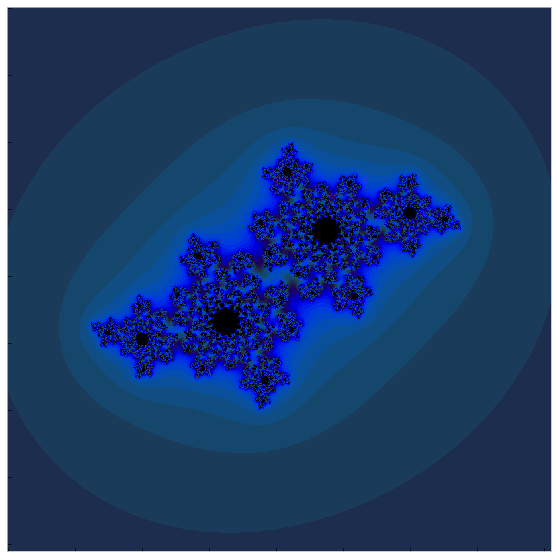# Introduction

After learning more about Tensorflow in class the other day, I decided it would be really easy and fun to revisit some of the work I did with fractals in a previous post but using the excellent Tensorflow library instead. This will be an incredibly short post.

As I learned, tensorflow can be useful for more than just constructing neural networks although that really is its primary purpose. It can be used for tensor operations of any kind…including things to do with fractals.

I should also note that this post was inspired by a particular lab exercise from my work at Galvanize.

# Some basic imports and setup

from __future__ import division, print_function

import matplotlib.pyplot as plt
import numpy as np
import tensorflow as tf

from matplotlib import animation
from matplotlib import colors

%matplotlib inline
plt.style.use('bmh')
c_map = plt.get_cmap('Dark2')


# Complex number plane

real_part = np.arange(start=-2, stop=2.05, step=0.005)
imag_part = np.arange(start=-2, stop=2.05, step=0.005)

X, Y = np.meshgrid(real_part, imag_part)
Z = X + 1j*Y


# Starting the tensorflow session

sess = tf.InteractiveSession()

zs = tf.Variable(Z, dtype=tf.complex64)

c = tf.constant(-0.4 + 0.6j, dtype=tf.complex64)
horizon = tf.constant(4., dtype=tf.float32)

ns = tf.Variable(np.zeros_like(Z), dtype=tf.int32)


# The meat of the problem

The Julia set is defined as the set of complex numbers that do not converge to a limit after a mapping is repeatedly applied. In this case the mapping I’ll use is the very common $f(z) = z^2 + C$ although in principle you could use any function.

I can define a tensorflow operation that will take z, calculated the next value of z and update, and test for convergence. Not only that–I can do that in one simple “group” in tensorflow.

step = tf.group(
)


# Run iterations

tf.global_variables_initializer().run()

for i in range(100):
step.run()


# Plot and enjoy!

def display_fractal(ns):
"""Display an array of iteration counts as a
colorful picture of a fractal."""
ns_cyclic = (6.28*ns/20.0).reshape(list(ns.shape) + )
color_channels = [10 + 20*np.cos(ns_cyclic),
30 + 50*np.sin(ns_cyclic),
155 - 80*np.cos(ns_cyclic)]
img = np.concatenate(color_channels, 2)
# Color the points that never escape black.
img[ns == ns.max()] = 0
# Keep the color channels between 0 and 255 (RGB).
img = np.uint8(np.clip(img, 0, 255))
return img

fig, ax = plt.subplots(figsize=(10, 10))

ax.imshow(display_fractal(ns.eval()));
ax.set_xticklabels('');
ax.set_yticklabels('');
ax.grid()